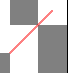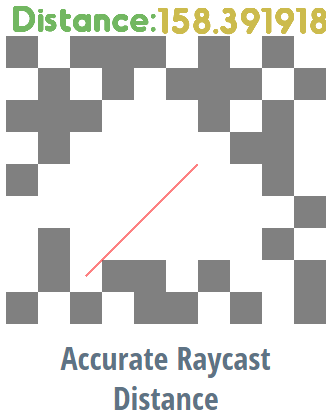# Accurate Raycast Hit Distance

Yes, this Raycast bundle outputs the actual, accurate distance to the target that’s being Hit.
HOWEVER, it only works for targets that have square hitboxes and cannot rotate at all (they can be movable, though.) So this works great for raycasting to walls or obstacles for instance.

Using the new “send to last Raycast target” message option, I figured out a way to calculate the precise distance to the target’s edge (not its center) using trigonometry.
Because this method uses the Message behavior, and due to a slight behavior order / timing delay that I can’t seem to find, the distance output has a tiny bit of lag (you can see this if you move the mouse around quickly—sometimes the ray appears to go through the wall for a frame before correcting to the proper length). Let me know if you have an idea on how to fix that and make the distance output more instant, as well as if you have any other feedback (or even an altogether better way of doing this).

10 Likes

Finally it’s here

Sounding like every youtube comment section.

1 Like

found an error (:1 Like

Hmm, I guess you could consider that an error, but in my opinion it’s kind of a matter of preference, whether you think raycasts should go through corners or not.
Whatever the case, though, the reason it goes through corners is because Flowlab’s raycast behavior (which I used as part of the code) allows rays at a perfect 45 degree angle to go through 2 blocks’ corners.

But thank you for the feedback still

6 Likes

This is pretty awesome,
I’m gonna look more into it later and see if I can put the expression together and try to find that delay.
But I have several ideas I want to try with this already lol.

4 LikesI also found something weird. Anyway it looks very smooth! I might want to try using this.

4 Likes

And got it, surprisingly this also fixes the delay as well.
I’m guessing the delay was because you were evaluating code with GridX and GridY separately.

Expression Code
``````var step0 = ((B%360)+360)%360;
var step1 = C>(E+A)?1:(C<(E-A)?1:0);
var step2 = Math.abs((Math.abs((Math.abs(E-C))-A))/(Math.cos((step0*Math.PI)/180)));
var step3 = ((Math.sin((step0*(Math.PI))/180))*step2)+D;
var step4 = step3>(F+A)?0:(step3<(F-A)?0:1);
var step5 = Math.abs((Math.abs((Math.abs(F-D))-A))/(Math.sin((step0*Math.PI)/180)));

step1==0? step5:step4 == 0? step5:step2

// A = 16 (Target Block Width/2)
// B = Angle
// C = this x
// D = this y
// E = Grid X
// F = Grid Y
``````
7 Likes

This is awesome you guys6 Likes

Fantastic, thanks for looking into it and improving it.
Do you want to make that code in a separate game or do you want me to just paste it into this one?
Edit: I created your bundle in the game to test it (it works) so let me know if you want me to remove it for you to put in your own example

3 Likes

Thanks, I’m glad you could see this (and JR01’s improvement).
It’s also worth mentioning that TinkerSmith accomplished something similar to this a while ago, but using a more performance-intensive method of repeatedly incrementing the raycast distance until it reaches the object edge . . . just wanted to list that here as well.
Anyways, maybe something like this could be implemented in the Raycast behavior itself in the future…?

3 Likes

Ah, ok that appears to be another bug; thanks for finding it.
Feel free to use my code however you want, though I guess you’ll have to ask JR01 about his version…

2 Likes

Nah, just use it.
I tried to replicate what you did all at once (each step is an expression). I wanted to make it where you could read the code and make your own adjustments if needed. Also it really cleans up the wires you had hehe.

2 Likes

Cool, thanks; yes, it’s a lot cleaner and I think I might even understand how your code works. I had no idea we can literally just declare variables and put them in expressions…that’s really useful.
Also if you happen to find a way around the triple absolute value that might clean things up even more XD

2 Likes

You can make a function for it, then declare that function to when you want to use it.
`function Abs(A) { Math.abs(A); } `

then you could do stuff like this:

``````function Abs(A) { Math.abs(A); }
function Ang(A) { ((A%360)+360)%360; }
function Sin(A) { Math.sin(A); }
function Cos(A) { Math.cos(A); }
var pi = Math.PI;

Abs((Abs((Abs(F-D))-A))/Sin((Ang(B)*pi)/180)))

//insteaf of
//Math.abs((Math.abs((Math.abs(F-D))-A))/(Math.sin(((((B%360)+360)%360)*Math.PI)/180)))
``````

I really suggest for anyone who wants to learn to also look into the code used for the ease bundle and how I put that all together.

5 Likes

Really cool that expressions allow you to make functions and create variables. Quick question, are functions/variables you create global between all expressions? I’m assuming it’s not, but just curious

2 Likes

Nope, you have to create these in all the expressions. It would be nice if Flowlab had a console in the main game settings to create global code.

3 Likes

This reminds me of Javascript, wait is flowlab.io made with javascript

1 Like

Flowlab is made with Javascript, Haxe, and HTML5.
Usually Haxe for coding inside the expressions.

3 Likes

I’ve finally fixed the bug with the corner hit outputting the wrong distance (at least I think it’s fixed).
I added some rounding to a part of the math that tests the y coordinate of the ray’s endpoint, which doesn’t affect the decimal accuracy of the raycast distance but seems to bypass some oddities in flowlab’s raycast behavior when hitting corners.
Anyways let me know if anyone finds a better way to solve that corner bug (I think the bug still happens if the blocks being targeted are movable, so any thoughts on that?)

5 Likes

i might have to mess around with this and maybe make my own lighting system or something else

2 Likes